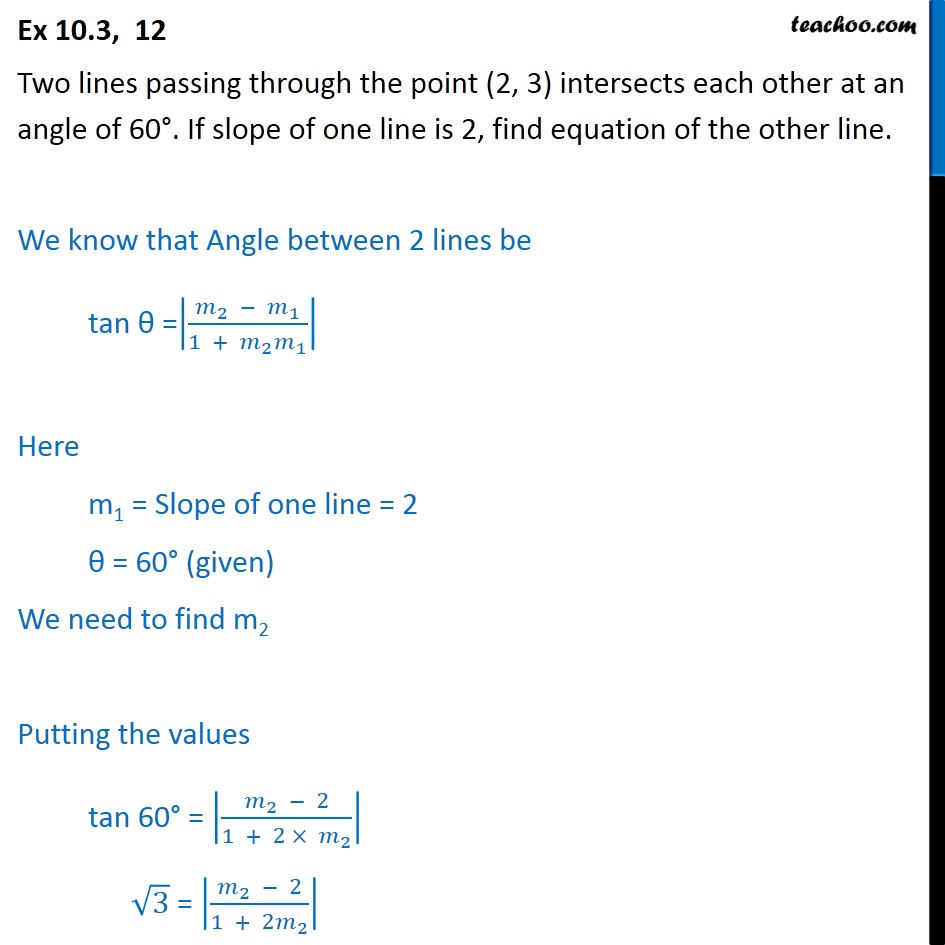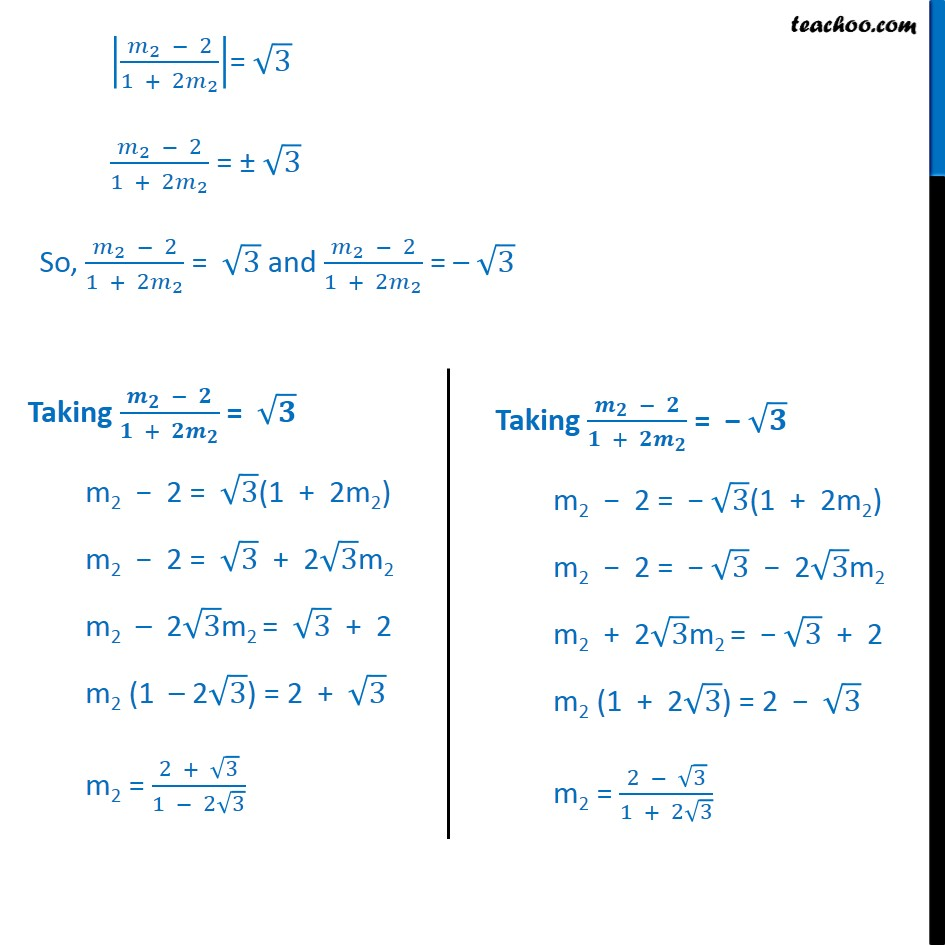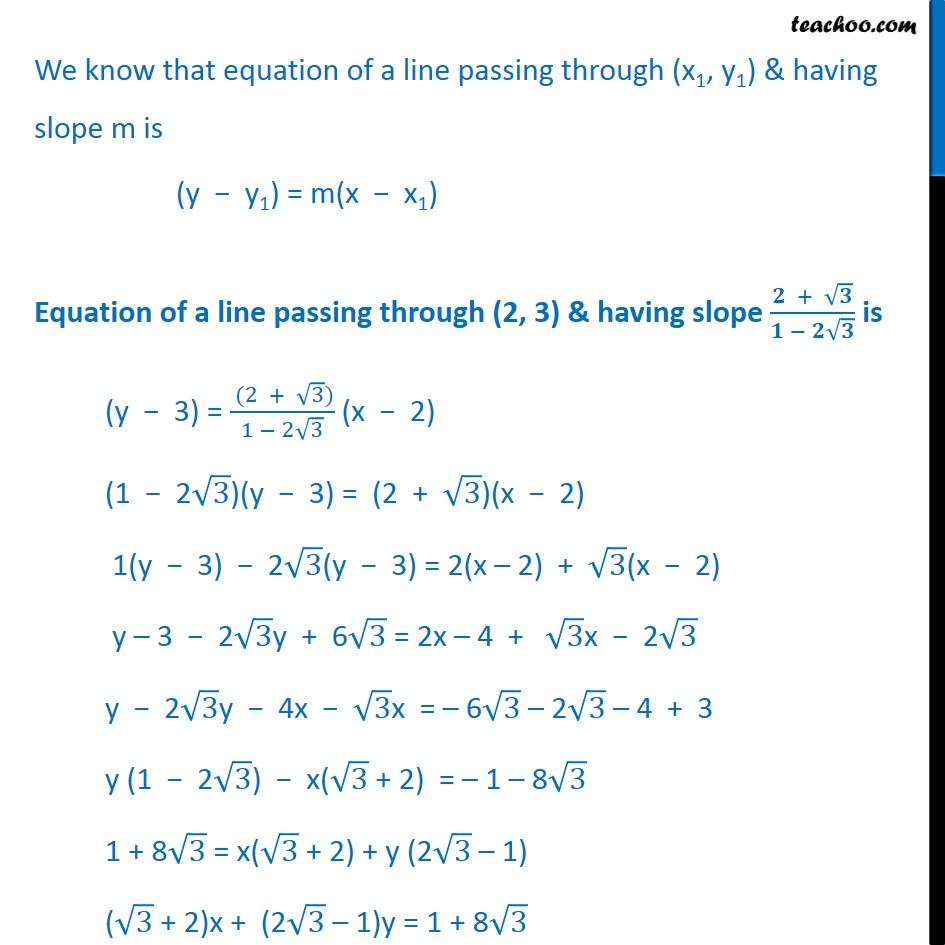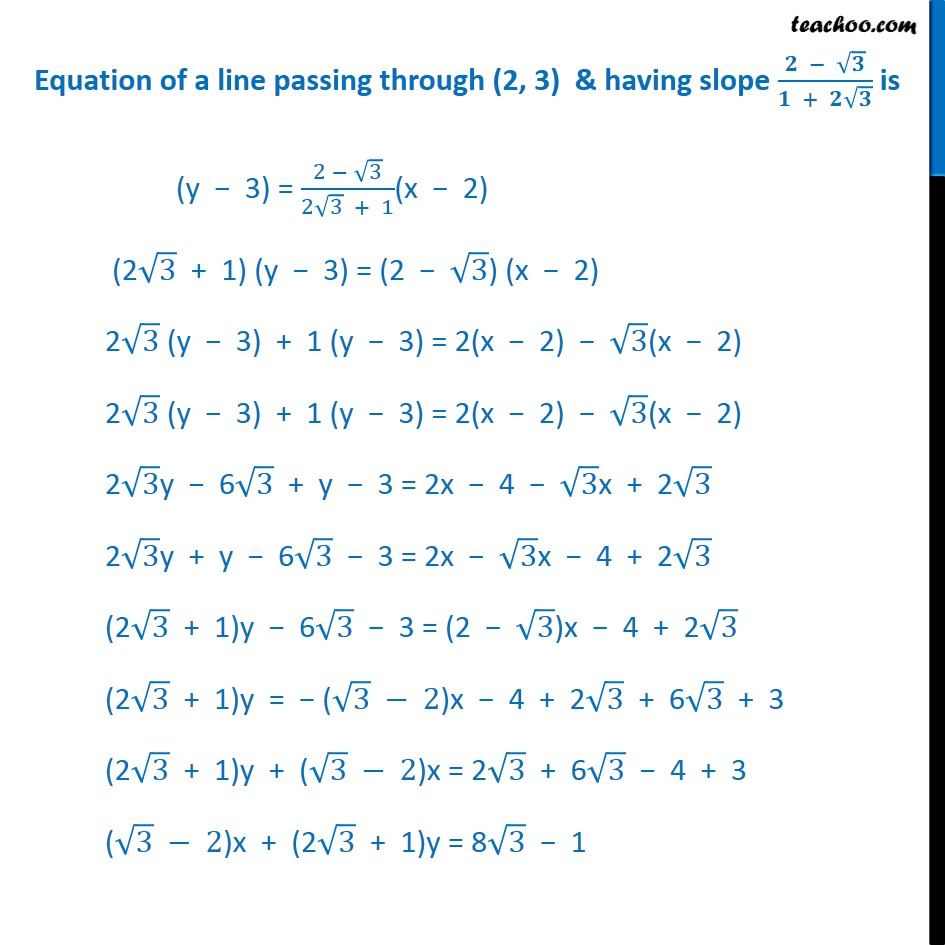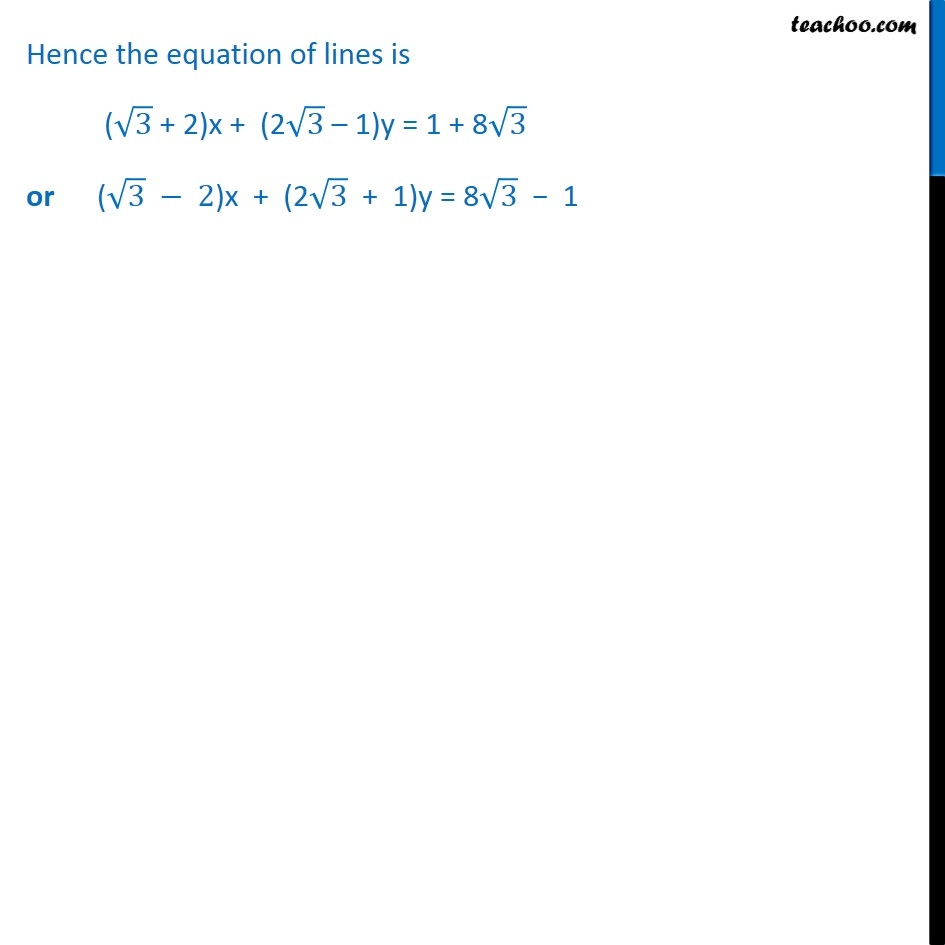1. Chapter 10 Class 11 Straight Lines
2. Serial order wise
3. Ex 10.3

Transcript

Ex 10.3, 12 Two lines passing through the point (2, 3) intersects each other at an angle of 60 . If slope of one line is 2, find equation of the other line. We know that Angle between 2 lines be tan =|( _2 _1)/(1 + _2 _1 )| Here m1 = Slope of one line = 2 = 60 (given) We need to find m2 Putting the values tan 60 = |( _2 2)/(1 + 2 _2 )| 3 = |( _2 2)/(1 + 2 _2 )| |( _2 2)/(1 + 2 _2 )|= 3 ( _2 2)/(1 + 2 _2 ) = 3 So, ( _2 2)/(1 + 2 _2 ) = 3 and ( _2 2)/(1 + 2 _2 ) = 3 We know that equation of a line passing through (x1, y1) & having slope m is (y y1) = m(x x1) Equation of a line passing through (2, 3) & having slope ( + )/( ) is (y 3) = ( (2 + 3))/(1 2 3) (x 2) (1 2 3)(y 3) = (2 + 3)(x 2) 1(y 3) 2 3(y 3) = 2(x 2) + 3(x 2) y 3 2 3y + 6 3 = 2x 4 + 3x 2 3 y 2 3y 4x 3x = 6 3 2 3 4 + 3 y (1 2 3) x( 3 + 2) = 1 8 3 1 + 8 3 = x( 3 + 2) + y (2 3 1) ( 3 + 2)x + (2 3 1)y = 1 + 8 3 Equation of a line passing through (2, 3) & having slope ( )/( + ) is (y 3) = (2 3)/(2 3 + 1)(x 2) (2 3 + 1) (y 3) = (2 3) (x 2) 2 3 (y 3) + 1 (y 3) = 2(x 2) 3(x 2) 2 3 (y 3) + 1 (y 3) = 2(x 2) 3(x 2) 2 3y 6 3 + y 3 = 2x 4 3x + 2 3 2 3y + y 6 3 3 = 2x 3x 4 + 2 3 (2 3 + 1)y 6 3 3 = (2 3)x 4 + 2 3 (2 3 + 1)y = ( 3 2)x 4 + 2 3 + 6 3 + 3 (2 3 + 1)y + ( 3 2)x = 2 3 + 6 3 4 + 3 ( 3 2)x + (2 3 + 1)y = 8 3 1 Hence the equation of lines is ( 3 + 2)x + (2 3 1)y = 1 + 8 3 or ( 3 2)x + (2 3 + 1)y = 8 3 1

Ex 10.3